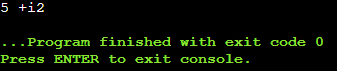Now let’s see how to overload the output stream operator that is ostream operator. We use cout for displaying some values on the screen and cin for reading some data from the keyboard. We can also overload cin and cout operators that are insertion and extraction operators.

In C++, stream insertion operator << is used for output and stean extraction operator >> is used for input. Before overloading these two operators, first, we should know the following things.

1. The cout is an object of ostream class and cin is an object of istream class
2. These two operators must be overloaded as a global function. And if we want these overloaded functions to access private data members of a class, then we must declare them as friend functions.
##### How to Overload Insertion Operator in C++?

Now, I am going to show you how to Overload Insertion Stream Operator in C++. So, let us take an example of a Complex class as follows:

```class Complex
{
private:
int real;
int img;
public:
Complex (int r = 0, int i = 0)
{
real = r;
img = i;
}
};
```

This is our Complex class. Here we have written two data members and one parameterized constructor. Now let us write the main function as follows.

```int main ()
{
Complex C1 (4, 3);
int x = 12;
cout << x;
cout << C1;
}
```

Here inside the main function, we have created an object C1 of the Complex class with values 4 and 3 for the real and img data members. Next, we have created an integer type variable x of value 12. Then we are displaying the value of x on the console. In the same way, we also want to print the values of real and img of C1. Then we should write cout << C1.

Does C++ know how to display complex numbers? No. Does it know how to display char, int, float, or double? Yes, any type of inbuilt data you can use with cout or with cin. If we write cout << C1; then this statement will give an error of “No match of operator”. Then how about displaying our own objects?

##### How to display our own Objects in C++?

We want to display values of C1 with the help of cout. The result should be ‘4 + 3i’ on the screen. We want the output like ‘4 + 3i’. C++ doesn’t know how to display a complex number. So, can we overload the insertion operator ‘<<’? Yes, we can overload the Stream Insertion Operator in C++.

Now let us write a function first that is for displaying real and img and then we will convert this function into an operator. The following Display function displays the real and img on the console window.

void Display(){
cout << real << ” + i” << img;
}

The above Display function will display a complex number in the format of ‘3 + 4i’. Now we will call the Display function from the main function as follows.

int main ()
{
Complex C1 (4, 3);
C1.Display();
}

The C1.Display(); statement will display ‘4 + 3i’ on the screen. So, in this way, we will display the complex number.

But we don’t want to call the Display function. We want C1 to be displayed like cout << C1. For this, we have to overload the insertion operator. So let us build the prototype.

First, instead of writing function name Display, we have to write operator <<. Then this insertion operator will take one or more parameters. What are the parameters? It will take an object of Complex as well as ostream object. So, it is taking two objects as parameters. So, we have to pass two objects as parameters as follows.

operator << (ostream &o, complex &c)

If you notice, we have passed objects by reference. Then after displaying the values, we want it to return back to the cout so that it can be used again in the same line. So instead of void, we have to write ostream& as the return type as follows.

ostream& operator << (ostream &o, Complex &c);

So, this is the signature. Now one more important point is, as this function is taking two parameters from two different types of objects, it cannot belong to the Complex class. So, we have to make this function a friend function. Below is the complete function signature for overloading the Insertion Operator in C++.

friend ostream& operator << (ostream &o, Complex &c);

This is a friend function and it is returning an output stream reference. As it is a friend function. It cannot be implemented inside the class. So, we have to provide the implementation of this function outside the class as follows:

```class Complex
{
private:
int real;
int img;
public:
Complex (int r = 0, int i = 0)
{
real = r;
img = i;
}
friend ostream& operator << (ostream& o, Complex& c);
};

ostream& operator << (ostream & o, Complex & c)
{
o << c.real << " +i" << c.img;
return o;
}
```

So, this is our class with the overloaded insertion operator in C++. Now let us write the complete program for this one.

```#include <iostream>
using namespace std;

class Complex
{
private:
int real;
int img;
public:
Complex (int r = 0, int i = 0)
{
real = r;
img = i;
}
friend ostream& operator << (ostream& o, Complex& c);
};

ostream& operator << (ostream & o, Complex & c)
{
o << c.real << " +i" << c.img;
return o;
}

int main()
{
Complex C1 (5, 2);
cout << C1;
}
```
###### Output: